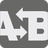Compare Functions
Elementary+
English

Okay, I guess we have to turn to plan B.
What's plan B?
Why wasn't that plan A?

- The Big Bang Theory : "The Lizard-Spock Expansion"

doveryai no proveryai (trust, but verify)

- Russian proverb adopted as signature phrase by Ronald Reagan

Two functions f and g are provided as inputs to checkio . The first function f is the primary function and the second function g is the backup. Use your coding skills to return a third function h which returns the same output as f unless f raises an exception or returns None . In this case h should return the same output as g . If both f and g raise exceptions or return None , then h should return None .

As a second output, h should return a status string indicating whether the function values are the same and if either function erred. A function errs if it raises an exception or returns a null value ( None ).

The status string should be set to: "same" if f and g return the same output and neither errs, "different" if f and g return different outputs and neither errs, "f_error" if f errs but not g , "g_error" if g errs but not f , or "both_error" if both err.

Input: Two functions: f (primary) and g (backup).

Output: A function h which takes arbitrary inputs and returns a two-tuple.

Example:

```        f = lambda x,y: x+y
g = lambda x,y: (x**2 - y**2)/(x-y)
checkio(f,g)(1,3) == (4,"same")
checkio(f,g)(1,1.01) == (2.01,"different") # numerical precision difference
checkio(f,g)(1,1) == (2,"g_error") # g divides by zero```

How it is used: This is an exercise in working with functions as first class objects.

Precondition: hasattr(f,'__call__');
hasattr(g,'__call__')